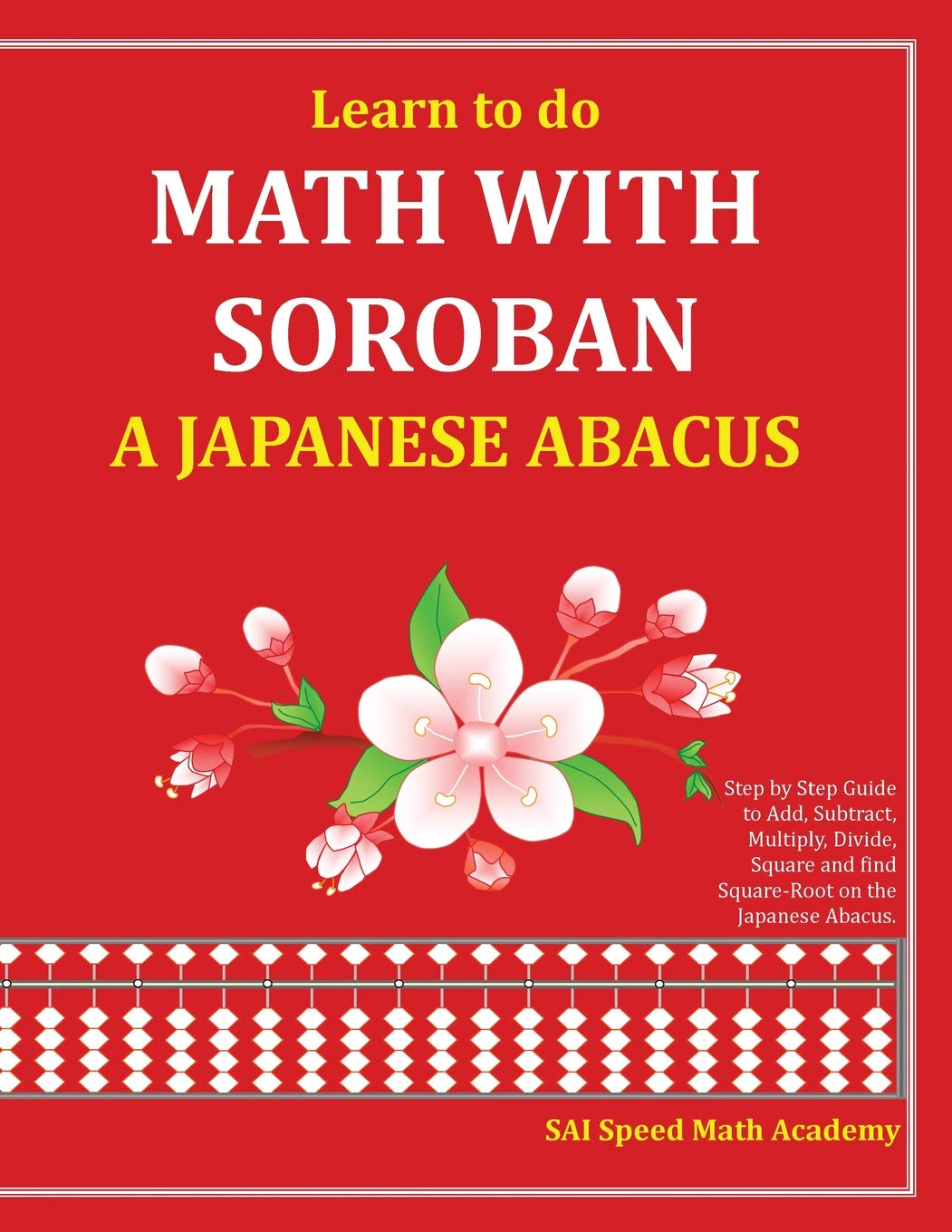# Learn to do Math With Soroban a Japanese Abacus: Learn how to add, subtract, multiply, divide and find square roots with this easy to use instruction guide.

Price: points - Details)

Learn how to add, subtract, multiply, divide and find square roots with this easy to use instruction guide. There are many sample problems with step-by-step instructions. The illustrations make it easy to follow along with the descriptions. Practice and perfect skills as you learn with the numerous practice problems given at the end of each chapter.Learn to do Math With Soroban a Japanese Abacus: Learn how to add, subtract, multiply, divide and find square roots with this easy to use instruction guide.